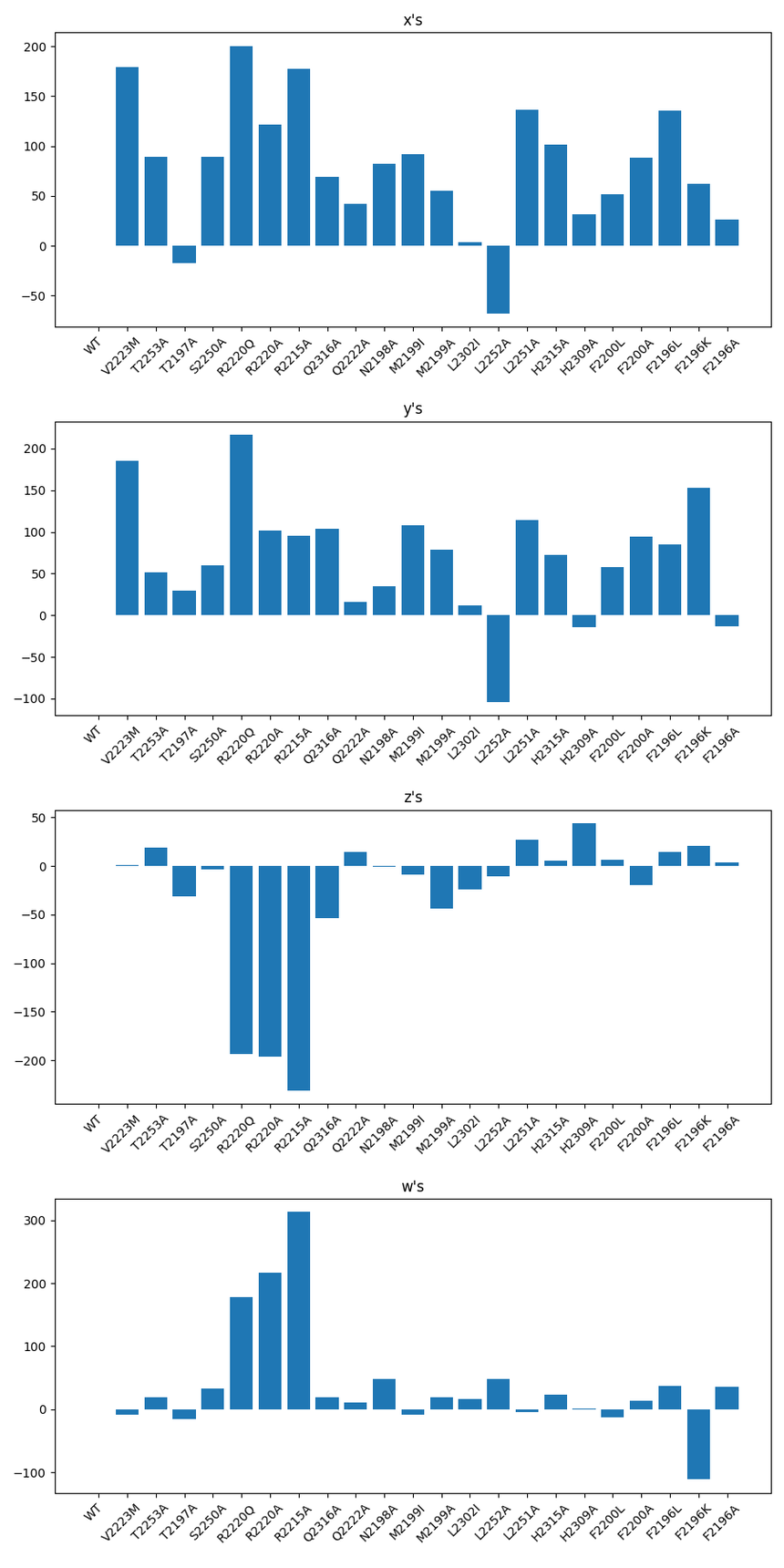Visualization technique for equations like w = x - y - z

Dear all,

I calculate energy values w like this:

w_1 = x_1 - y_1 - z_1

....

w_18 = x_18 - y_18 - z_18

I am trying to find a good vizualization technique that shows the influence of the x's, y's and z's on the w's. I want to make statements like: 'In case w_5, x is especially high but y is too which is why w is not so high' or 'z in case w_9 is negative whereas y is positive which is why w is almost only determined by the magnitude of x'.

A simple bar chart of the 4 components is hard to interpret, at least for me. I thought about pie charts, stacked bar charts and 3D visualizations but did not find the right visualization technique yet. I attached the data if you want to have a go at it (all values in the bar chart are relative to the value of WT, which is why WT is 0 in all bar charts).

Looking forward to your ideas and I am happy to provide further information.

cheers

Martin